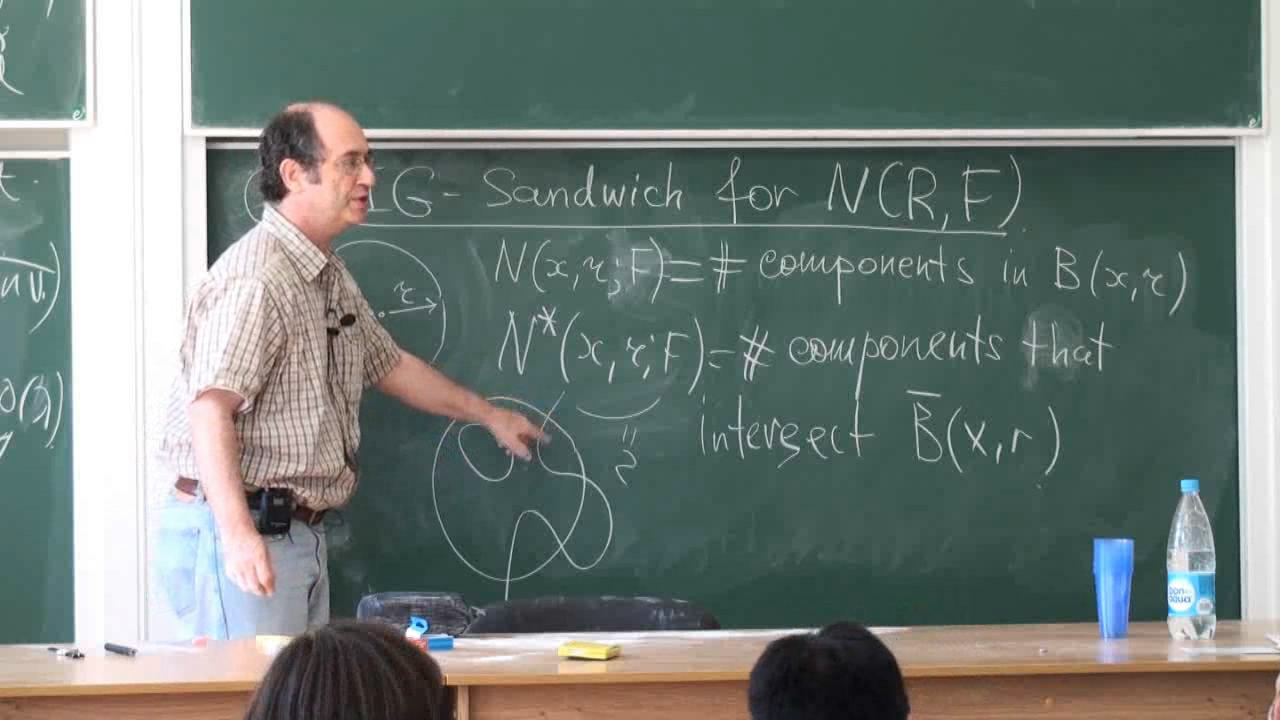# Random nodal portraits

Курс Хит
Предмет:We describe the progress and challenges of understanding the zero sets of smooth Gaussian random functions of several real variables. The primary examples are various ensembles of Gaussian real-valued polynomials (algebraic or trigonometric) of large degree, and smooth Gaussian functions on the Euclidean space with translation-invariant distribution.

We start with an intriguing heuristics suggested by Bogomolny and Schmit, which relates nodal portraits of 2D Gaussian monochromatic waves to bond percolation on the square lattice. Then we explain how tools from classical analysis (Gaussian isoperimetric inequality proved by Sudakov-Tsirelson and Borell, and Wiener's ergodic theorem) help to find the order of growth of the typical number of connected components of the zero set. This might be thought as a statistical version of Hilbert's 16th problem.

The lectures are based on joint works with Fedor Nazarov.

Курс прочитан в рамках St. Petersburg School in Probability and Statistical Physics

3